# Chapter 11. The Tilt Intonation Model

Theoretical Overview
Exectuable Programs
Tilt functions

Tilt is a phonetic model of intonation that represents intonation as a sequence of continuously parameterised events. The tilt library is a set of functions which analyses, synthesizes and manipulates tilt representations.

# Theoretical Overview

The basic unit in the tilt model is the intonational event. Events occur as instants with nothing between them, as opposed to segmental based phenomena where units occur in a contiguous sequence. The basic types of intonational event are pitch accents and (following the popular terminology) boundary tones. Pitch accents (denoted by the letter a) are F0 excursions associated with syllables which are used by the speaker to give some degree of emphasis to a particular word or syllable. In the tilt model, boundary tones (b) are rising F0 excursions which occur at the edges of intonational phrases and as well as giving the hearer a cue as to the end of the phrase, can also signal effects such as continuation and questioning. A combination event ab occurs when a pitch accent and boundary tone occur so close to one another that only a single pitch movement is observed. There are different kinds of pitch accents and boundary tones: the choice of pitch accent and boundary tone allows the speaker to produce different global intonational tunes which can indicate questions, statements, moods etc to the hearer.

Figure 11-1.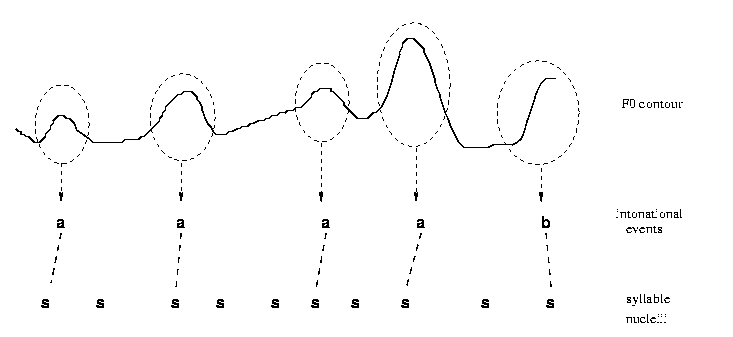Figure 11-1 shows a Schematic representation of F0, intonational event relation and segment relation in the Tilt model. The linguistically relevant parts of the F0 contour, which correspond to intonational events, are circled. The events, labelled a for pitch accent and b for boundary are linked to the syllable nuclei of the syllable relation. Note that every event is linked to a syllable, but some syllables do not have events.

Unlike traditional intonational phonology schemes \cite{ph:thesis}, \cite{tobi} which impose a categorical classification on events, Tilt uses a set of continuous parameters. These parameters, collectively known as tilt parameters, are determined from examination of the local shape of the event's F0 contour.

The tilt model is built on a simpler model, the rise/fall/connection (RFC) model.

In the RFC model, each event is modelled by a rise part followed by a fall part. Each part has an amplitude and duration, and two parameters are used to give the time position of the event in the utterance and the F0 height of the event. Figure 11-2 shows a typical pitch accent with these parameters marked.

Figure 11-2.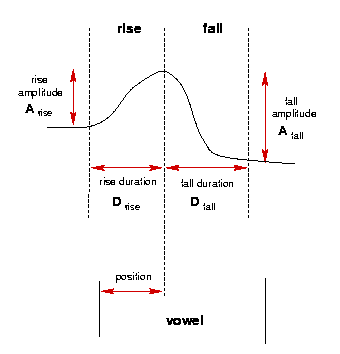The RFC parameters for an utterance are therefore:

• rise amplitude (Hz)

• rise duration (seconds)

• fall amplitude (Hz)

• fall duration (seconds)

• position (seconds)

• F0 height (Hz)

Sometimes events don't have rise or fall parts, and in these cases the amplitude and duration of the missing part is set to 0. The position parameter can be specified in two ways: either as the distance from the start of the utterance, or the distance from the start of the vowel of the associated syllable. The latter is more linguistically meangingful, but as vowel boundaries are not always available, the former is often used.

While the RFC model can accurately describe F0 contours, the mechanism is not ideal in that the RFC parameters for each contour are not as easy to interpret and manipulate as one might like. For instance there are two amplitude parameters for each event, when it would make sense to have only one.

The Tilt representation helps solve these problems by transforming the four amplitude and duration RFC parameters into three Tilt parameters:

• amplitude (Hz): the sum of the magnitudes of the rise and fall amplitudes.

• duration (seconds): the sum of the rise and fall durations.

• tilt: a dimensionless number which expresses the overall shape of the event, independent of its amplitude or duration.

The position and F0 height parameters are the same as before.

The tilt representation is superior to the RFC representation in that it has fewer parameters without significant loss of accuracy. Importantly, it can be argued that the tilt parameters are more linguistically meaningful.

In describing the tilt model, we use the term analysis to describe the process of producing a tilt representation from an F0 contour, and synthesis to describe the process of prodcing a F0 contour from a tilt representation.

## RFC Analysis

### Locating Events in the F0 contour

The first stage in analysis is to find the intonational events in an F0 contour. EST does not directly provide a means for doing this. In practice this is either done by hand by a human labeller, or automatically by the HMM auto event labeller. The current HMM event labeller is based on the HTK system and hence can't be part of EST, but an outline of the system follows:

The automatic event detector uses continuous density hidden Markov models to perform a segmentation of the input utterance. A number of units are defined and a HMM is trained on examples of that kind from a pre-labelled training corpus using the Baum-Welch algorithm \cite{baum:72}. Each utterance in the corpus is acoustically processed so that it can be represented by sequence of evenly spaced frames. Each frame is a multi-component vector representing the acoustic information for the time interval centred around the frame.

Recognition is performed by forming a network comprising the HMMs for each unit in conjunction with an n-gram language model which gives the prior probability of a sequence of n units occurring. To perform recognition on an utterance, the network is searched using the standard Viterbi algorithm to find the most likely path through the network given the input sequence of acoustic vectors.

It is our intention to put a complete event labeller in EST in the future.

### Producing an RFC representation from an utterance's events and F0 contour

An utterance's events are represented in a relation. Initially, events are stored as regions with start and stop times as this is the most common output format of labellers (both human and automatic).

For example, for utterance kdt_016, a set of basic event labels is as follows (in xlabel format):

 0.290 146 sil 0.480 146 c 0.620 146 a 0.760 146 c 0.960 146 a 1.480 146 c 1.680 146 a 1.790 146 sil

Events are labelled "a", and silences "sil". The use of the "c" label is to allow start times which differ from the end of the previous event. Conceptually, this can alsow be represented as follows:

 name:sil start:0.0 end:0.290 name:a start:0.290 end:0.620 name:a start:0.760 end:0.960 name:a start:1.480 end:1.680 name:sil start:1.790 end:1.790

The other component for analysis is the utterance's F0 contour, which is stored in a track. The contour must be continuous (i.e. have no breaks), and its frames must be specified at fixed intervals. For best performance the contour should have been smoothed.

The RFC analysis component takes the approximate labels and the smoothed F0 contour, fits rise and fall shapes, and hence determines an optimal set of RFC parameters for the utterance.

For each event, a peak picking algorithm decides if the event has a rise part only, a fall part only or a rise part followed by a fall part.

Figure 11-3.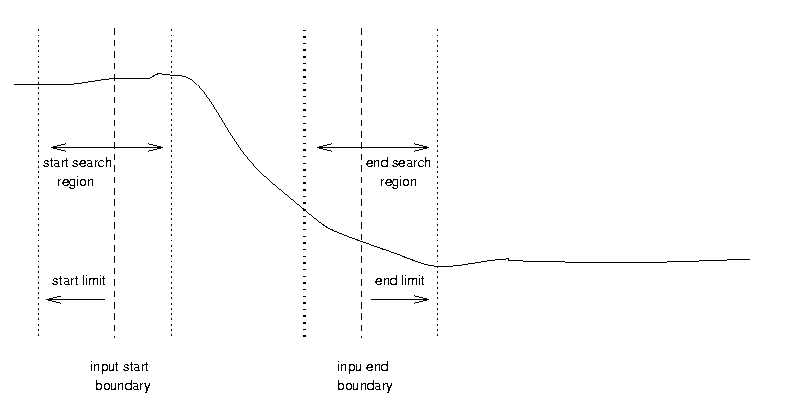For each part, a search region, shown in Figure 11-3, is defined around the approximate start and end boundaries as defined in the input label file. The search region is controlled by a number of parameters:

• start_limit: the distance in seconds before each input start boundary that the start search region should begin.

• end_limit: the distance in seconds after each input end boundary that the end search region should begin.

• range: the end and beginnings of the start and end regions respectively, specified as a fraction of the overall label duration.

For example, a pitch accent starts at 1.45 seconds and ends at 1.75 seconds. If the start and end limit are both defined to be 0.1 seconds and the range is 0.4 (40%), then the start region starts at 1.35 seconds and ends at 1.55, and the end region starts at 1.65 and ends at 1.85. The matching algroithm will synthesize every possible shape lying within this region, measure the distance between each and the actual contour, and pick the one with the lowest distance.

The final results of the matching process is a relation of events, each with the 6 RFC parameters are descibed above.

The program tilt_analysis will perform RFC matching given a label file and F0 contour. The function rfc_analysis takes a F0 contour, a relation and a set of options and returns the RFC parameters in the features of each item in the relation.

## RFC to Tilt Conversion

The rise and fall RFC parameters can be converted to Tilt parameters using the following equations. Amplitude is the sum of te magnitudes of the rise and fall amplitudes:

Equation 11-1.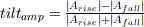Duration is the sum of the of the rise and fall durations:

Equation 11-2.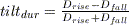Tilt can be measured with respect to amplitude:

Equation 11-3.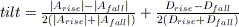or duration:

Equation 11-4.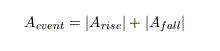The tilt model assumes that these are strongly correlated so that an average of the two is representative of the shape of the event:

Equation 11-5.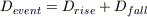The is no stand alone program to do this conversion, but the tilt_analysis can do this conversion in addition to performing the RFC matching as described above.

The function rfc_to_tilt takes a relation containing RFC parameterised items and converts it to a relation containing Tilt paramterised items.

Another function, also called rfc_to_tilt takes a Features object containing the 4 rise fall paramaters and writes the 3 tilt paramaters into another features object. This function can be used to do rfc_to_tilt conversion for a single event.

## Tilt to RFC Conversion

The Tilt parameters can be converted to RFC parameters using the follwing equations, which are rearranged from Equation 11-1 to Equation 11-5:

Equation 11-6. Rise amplitude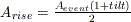Equation 11-7. Fall amplitude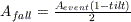Equation 11-8. Rise duration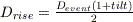Equation 11-9. Fall duration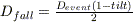The is no stand alone program to do this conversion, but the tilt_synthesis can do this conversion in addition to generating a F0 contour.

The function tilt_to_rfc takes a relation containing Tilt parameterised items and converts it to a relation containing RFC paramterised items.

Another function, also called tilt_to_rfc takes a Features object containing the 3 Tilt paramaters and writes the 4 rise fall RFC paramaters into another features object. This function can be used to do tilt_to_rfc conversion for a single event.

## RFC to F0 Synthesis

An F0 contour can be generated from a set of RFC parameters using the follwing equations. Events are generated as piecewise combinations of quadratic functions:

Equation 11-10.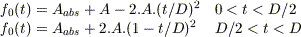Between events, straight lines are used:

Equation 11-11.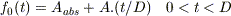The stand alone program tilt_synthesis can do this conversion. It takes a RFC label file as input and produces a F0 file. This program can also generate a F0 file directly from a Tilt label file

The function rfc_synthesis takes a relation containing RFC parameterised items and produces a F0 contour in a Track.

The function synthesize_rf_event takes a Features object containing the 4 rise fall RFC paramaters and generates the F0 contour for a single event.